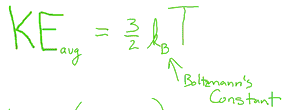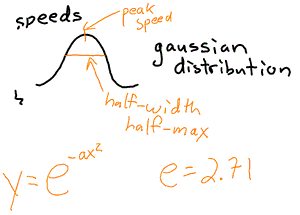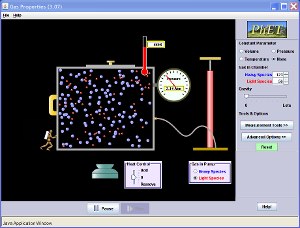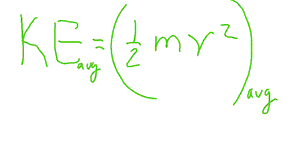## Temperature

“Ole Roemer created the first commonly used temperature scale in 1702 in Denmark (The Roemer scale). Roemer used a thermometer containing alcohol with a red dye. He created his scale by dividing the temperature between the freezing and boiling points of water into 80 degrees.

Gabriel Fahrenheit, a German physicist, was the first man to use a mercury thermometer.  In 1714, in Holland, he developed the Fahrenheit scale.  However, he did not base his scale on the freezing and boiling points of water. Instead, for his zero point he used the lowest temperature he could create in the laboratory from a mixture of water, salt, and ice. For the other end of his scale he used the temperature of the human body for 100 degrees.  Eventually the Fahrenheit scale was redesigned so that water freezes at 32 degrees, water boils at 212 degrees, and the scale between freezing and boiling is divided into 180 degrees.

In 1742 Anders Celsius, a Swedish astronomer invented a scale in which there were 100 degrees between freezing and boiling.  This is the centigrade scale. In 1948 the official scale of the metric system was named the Celsius scale in honor of Anders Celsius.”

I wrote the above for a textbook I worked on back in the late 90’s.  Save to say, there are some temperature scales you have not heard of before.  However, if you look up Roemer on Wikipedia, you will find some interesting discussions of the Rømer scale and which is very different from the Réaumur scale. So why was I so misinformed back in the late 90s, besides not having Wikipedia.  Interestingly, I have some very old textbooks with unit conversions between Fahrenheit, Celsius, and Reaumer scales.  So if you were in school 100 years ago, you would have a lot more temperature conversions to learn! It was used commonly in southern Europe (and is still used for making cheese).

In middle school you learned your unit conversions from °F to °C.  Hopefully, you also did some unit conversions with the Kelvin Scale, or the absolute zero scale.  To convert from Kelvin to degrees Celsius, you just add 273 °C.

So the freezing point of water is at  273 K = 0 °C = 32 °F, and the boiling point of water is at 373 K = 100 °C = 212 °F

Note that Kelvin temperatures do not use the degree symbol.  If you look at thermodynamic engineering texts, they use a different absolute value scale, the Rankine Scale.

But what is temperature?  In elementary school, you learned it was how cold or hot things are.

We can define temperature as proportional to the average kinetic energy per molecule. This temperature would be measured in the Kelvin scale. The k is Boltzmann’s constant who did a lot of early pioneering work on understanding thermodynamics using a branch of physics known as Statistical Mechanics.Thus with this explanation of temperature, using the Kelvin scale, temperature is a measure of the motion.

Note that the key word in the equation is that temperature is a measurement of the AVERAGE energy.

If we look at a distribution of the speeds of the molecules in a gas, we find that it forms a Gaussian curve.  This curve is commonly found in mathematical distributions for random numbers and noise.  We can characterize the Gaussian distribution of speeds by looking at the peak speed and the half-width, half-max of the distribution of speeds.You can examine these speeds by playing around with the PHET simultion and looking at the histograms of speeds.Click to Run

Interestingly, you should notice what happens to the distribution of speeds when you use light molecules versus heavy molecules.

﻿Think about the equation for kinetic energy. How will these distributions change?Two gasses of different masses when mixed will have the same temperature, but the speed distributions will be different.